FFT_fft - CSDN

• FFT是离散傅立叶变换的快速算法，可以将一个信号变换到频域。有些信号在时域上是很难看出什么特征的，但是如果变换到频域之后，就很容易看出特征了。这就是很多信号分析采用FFT变换的原因。另外，FFT可以将一个信号...
转自：https://blog.csdn.net/zhaopeizhaopeipei/article/details/53908238

FFT是离散傅立叶变换的快速算法，可以将一个信号变换到频域。有些信号在时域上是很难看出什么特征的，但是如果变换到频域之后，就很容易看出特征了。这就是很多信号分析采用FFT变换的原因。另外，FFT可以将一个信号的频谱提取出来，这在频谱分析方面也是经常用的。

虽然很多人都知道FFT是什么，可以用来做什么，怎么去做，但是却不知道FFT之后的结果是什意思、如何决定要使用多少点来做FFT。
采样得到的数字信号，就可以做FFT变换了。N个采样点，经过FFT之后，就可以得到N个点的FFT结果。为了方便进行FFT运算，通常N取2的整数次方。
假设采样频率为Fs，信号频率F，采样点数为N。那么FFT之后结果就是一个为N点的复数。每一个点就对应着一个频率点。这个点的模值，就是该频率值下的幅度特性。具体跟原始信号的幅度有什么关系呢？假设原始信号的峰值为A，那么FFT的结果的每个点（除了第一个点直流分量之外）的模值就是A的N/2倍。而第一个点就是直流分量，它的模值就是直流分量的N倍。而每个点的相位呢，就是在该频率下的信号的相位。第一个点表示直流分量（即0Hz），而最后一个点N的再下一个点（实际上这个点是不存在的，这里是假设的第N+1个点，也可以看做是将第一个点分做两半分，另一半移到最后）则表示采样频率Fs，这中间被N-1个点平均分成N等份，每个点的频率依次增加。例如某点n所表示的频率为：Fn=(n-1)*Fs/N。由上面的公式可以看出，Fn所能分辨到频率为为Fs/N，如果采样频率Fs为1024Hz，采样点数为1024点，则可以分辨到1Hz。1024Hz的采样率采样1024点，刚好是1秒，也就是说，采样1秒时间的信号并做FFT，则结果可以分析到1Hz，如果采样2秒时间的信号并做FFT，则结果可以分析到0.5Hz。如果要提高频率
分辨力，则必须增加采样点数，也即采样时间。频率分辨率和采样时间是倒数关系。
假设FFT之后某点n用复数a+bi表示，那么这个复数的模就是An=根号a*a+b*b，相位就是Pn=atan2(b,a)。根据以上的结果，就可以计算出n点（n≠1，且n<=N/2）对应的信号的表达式为：An/(N/2)*cos(2*pi*Fn*t+Pn)，即2*An/N*cos(2*pi*Fn*t+Pn)。对于n=1点的信号，是直流分量，幅度即为A1/N。
由于FFT结果的对称性，通常我们只使用前半部分的结果，即小于采样频率一半的结果。
好了，说了半天，看着公式也晕，下面圈圈以一个实际的信号来做说明。
假设我们有一个信号，它含有2V的直流分量，频率为50Hz、相位为-30度、幅度为3V的交流信号，以及一个频率为75Hz、相位为90度、幅度为1.5V的交流信号。用数学表达式就是如下：
S=2+3*cos(2*pi*50*t-pi*30/180)+1.5*cos(2*pi*75*t+pi*90/180)
式中cos参数为弧度，所以-30度和90度要分别换算成弧度。我们以256Hz的采样率对这个信号进行采样，总共采样256点。按照我们上面的分析，Fn=(n-1)*Fs/N，我们可以知道，每两个点之间的间距就是1Hz，第n个点的频率就是n-1。我们的信号有3个频率：0Hz、50Hz、75Hz，应该分别在第1个点、第51个点、第76个点上出现峰值，其它各点应该接近0。实际情况如何呢？我们来看看FFT的结果的模值如图所示。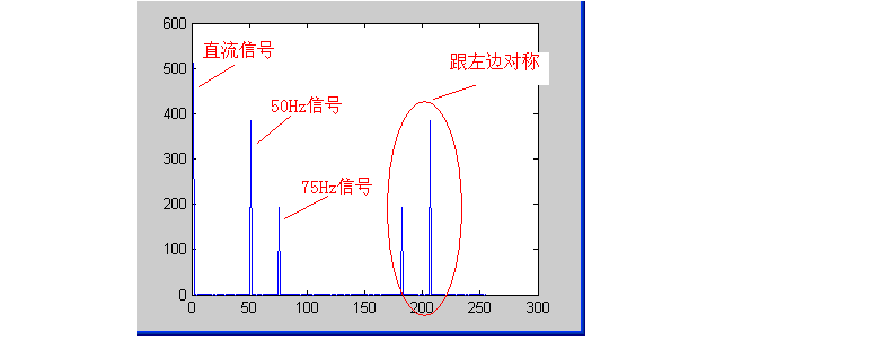图1 FFT结果
从图中我们可以看到，在第1点、第51点、和第76点附近有比较大的值。我们分别将这三个点附近的数据拿上来细看：
1点： 512+0i
2点： -2.6195E-14 - 1.4162E-13i
3点： -2.8586E-14 - 1.1898E-13i
50点：-6.2076E-13 - 2.1713E-12i
51点：332.55 - 192i
52点：-1.6707E-12 - 1.5241E-12i
75点：-2.2199E-13 -1.0076E-12i
76点：3.4315E-12 + 192i
77点：-3.0263E-14 +7.5609E-13i

很明显，1点、51点、76点的值都比较大，它附近的点值都很小，可以认为是0，即在那些频率点上的信号幅度为0。接着，我们来计算各点的幅度值。分别计算这三个点的模值，结果如下：
1点： 512
51点：384
76点：192
按照公式，可以计算出直流分量为：512/N=512/256=2；50Hz信号的幅度为：384/(N/2)=384/(256/2)=3；75Hz信号的幅度为192/(N/2)=192/(256/2)=1.5。可见，从频谱分析出来的幅度是正确的。
然后再来计算相位信息。直流信号没有相位可言，不用管它。先计算50Hz信号的相位，atan2(-192, 332.55)=-0.5236,结果是弧度，换算为角度就是180*(-0.5236)/pi=-30.0001。再计算75Hz信号的相位，atan2(192, 3.4315E-12)=1.5708弧度，换算成角度就是180*1.5708/pi=90.0002。可见，相位也是对的。
根据FFT结果以及上面的分析计算，我们就可以写出信号的表达式了，它就是我们开始提供的信号。
总结：假设采样频率为Fs，采样点数为N，做FFT之后，某一点n（n从1开始）表示的频率为：Fn=(n-1)*Fs/N；该点的模值除以N/2就是对应该频率下的信号的幅度（对于直流信号是除以N）；该点的相位即是对应该频率下的信号的相位。相位的计算可用函数atan2(b,a)计算。atan2(b,a)是求坐标为(a,b)点的角度值，范围从-pi到pi。要精确到xHz，则需要采样长度为1/x秒的信号，并做FFT。要提高频率分辨率，就需要增加采样点数，这在一些实际的应用中是不现实的，需要在较短的时间内完成分析。解决这个问题的方法有频率细分法，比较简单的方法是采样比较短时间的信号，然后在后面补充一定数量的0，使其长度达到需要的点数，再做FFT，这在一定程度上能够提高频率分辨力。具体的频率细分法可参考相关文献。
[附录：本测试数据使用的matlab程序]
close all; %先关闭所有图片
A1=3;   %频率F1信号的幅度
A2=1.5; %频率F2信号的幅度
F1=50;  %信号1频率(Hz)
F2=75;  %信号2频率(Hz)
Fs=256; %采样频率(Hz)
P1=-30; %信号1相位(度)
P2=90;  %信号相位(度)
N=256;  %采样点数
t=[0:1/Fs:N/Fs]; %采样时刻
%信号
%显示原始信号
plot(S);
title('原始信号');
figure;
Y = fft(S,N); %做FFT变换
Ayy = (abs(Y)); %取模
plot(Ayy(1:N)); %显示原始的FFT模值结果
title('FFT 模值');
figure;
Ayy=Ayy/(N/2);   %换算成实际的幅度
Ayy(1)=Ayy(1)/2;
F=([1:N]-1)*Fs/N; %换算成实际的频率值
plot(F(1:N/2),Ayy(1:N/2));   %显示换算后的FFT模值结果
title('幅度-频率曲线图');
figure;
Pyy=[1:N/2];
for i="1:N/2"
Pyy(i)=phase(Y(i)); %计算相位
Pyy(i)=Pyy(i)*180/pi; %换算为角度
end;
plot(F(1:N/2),Pyy(1:N/2));   %显示相位图
title('相位-频率曲线图');
看完这个你就明白谐波分析了。

展开全文• FFT有什么用 快速傅里叶变换 (fast Fourier transform),即利用计算机计算离散傅里叶变换（DFT)的高效、快速计算方法的统称，简称FFT。快速傅里叶变换是1965年由J.W.库利和T.W.图基提出的。采用这种算法能使...
FFT前言

快速傅里叶变换 (fast Fourier transform),即利用计算机计算离散傅里叶变换（DFT)的高效、快速计算方法的统称，简称FFT。快速傅里叶变换是1965年由J.W.库利和T.W.图基提出的。采用这种算法能使计算机计算离散傅里叶变换所需要的乘法次数大为减少，特别是被变换的抽样点数N越多，FFT算法计算量的节省就越显著。
FFT（Fast Fourier Transformation） 是离散傅氏变换（DFT）的快速算法。即为快速傅氏变换。它是根据离散傅氏变换的奇、偶、虚、实等特性，对离散傅立叶变换的算法进行改进获得的。
——百度百科

FFT（Fast Fourier Transformation），中文名快速傅里叶变换，用来加速多项式乘法
朴素高精度乘法时间$O(n^2)$，但$FFT$能$O(n\log_2 n)$的时间解决
$FFT$名字逼格高，也难懂，其他教程写得让人看不太懂，于是自己随便写一下

建议对复数、三角函数相关知识有所耳闻 （不会也无所谓）

下面难懂的点我会从网上盗

多项式的系数表示法和点值表示法

$FFT$其实是一个用$O(n\log_2n)$的时间将一个用系数表示的多项式转换成它的点值表示的算法

多项式的系数表示和点值表示可以互相转换

系数表示法

一个n-1次n项多项式$f(x)$可以表示为$f(x)=\sum^{n-1}_{i=0}a_ix^i$
也可以用每一项的系数来表示$f(x)$，即$f(x)=\{a_0,a_1,a_2,...,a_{n-1} \}$
这就是系数表示法，也就是平时数学课上用的方法

点值表示法

把多项式放到平面直角坐标系里面，看成一个函数

把$n$个不同的$x$代入，会得出$n$个不同的$y$，在坐标系内就是$n$个不同的点

那么这$n$个点唯一确定该多项式，也就是有且仅有一个多项式满足$∀k,f(x_k)=y_k$

理由很简单，把$n$条式子联立起来成为一个有n条方程的n元方程组，每一项的系数都可以解出来

那么$f(x)$还可以用$f(x)=\{(x_0,f(x_0)),(x_1,f(x_1)),(x_2,f(x_2)),...,(x_{n-1},f(x_{n-1}))\}$来表示
这就是点值表示法

高精度乘法下两种多项式表示法的区别
对于两个用系数表示的多项式，我们把它们相乘
设两个多项式分别为$A(x),B(x)$
我们要枚举$A$每一位的系数与$B$每一位的系数相乘
那么系数表示法做多项式乘法时间复杂度$O(n^2)$
但两个用点值表示的多项式相乘，只需要$O(n)$的时间

什么意思呢？

设两个点值多项式分别为$f(x)=\{(x_0,f(x_0)),(x_1,f(x_1)),(x_2,f(x_2)),...,(x_{n-1},f(x_{n-1}))\}$$g(x)=\{(x_0,g(x_0)),(x_1,g(x_1)),(x_2,g(x_2)),...,(x_{n-1},g(x_{n-1}))\}$
设它们的乘积是$h(x)$，那么$h(x)=\{(x_0,f(x_0)·g(x_0)),(x_1,f(x_1)·g(x_1)),...,(x_{n-1},f(x_{n-1})·g(x_{n-1}))\}$
所以这里的时间复杂度只有一个枚举的$O(n)$

突然感觉高精度乘法能$O(n)$暴艹一堆题？

但是朴素的系数表示法转点值表示法的算法还是$O(n^2)$的，逆操作类似

朴素系数转点值的算法叫DFT（离散傅里叶变换），点值转系数叫IDFT（离散傅里叶逆变换）

难道高精度乘法只能$O(n^2)$了吗？

DFT前置知识&技能

复数

毕竟高中有所以不多说

我们把形如a+bi（a,b均为实数）的数称为复数，其中a称为实部，b称为虚部，i称为虚数单位。当虚部等于零时，这个复数可以视为实数；当z的虚部不等于零时，实部等于零时，常称z为纯虚数。复数域是实数域的代数闭包，也即任何复系数多项式在复数域中总有根。 复数是由意大利米兰学者卡当在十六世纪首次引入，经过达朗贝尔、棣莫弗、欧拉、高斯等人的工作，此概念逐渐为数学家所接受。
——百度百科

初中数学老师会告诉你没有$\sqrt{-1}$，但仅限$R$
扩展至复数集$C$，定义$i^2=-1$，一个复数$z$可以表示为$z=a+bi(a,b\in R)$
其中$a$称为实部，$b$称为虚部，$i$称为虚数单位

在复数集中就可以用$i$表示负数的平方根，如$\sqrt{-7}=\sqrt{7}i$

还可以把复数看成复平面直角坐标系上的一个点，比如下面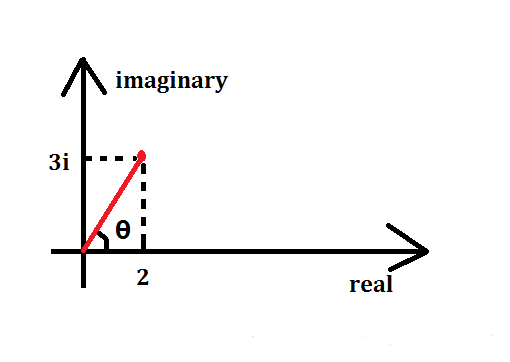$x$轴就是实数集中的坐标轴，$y$轴就是虚数单位$i$轴

这个点$(2,3)$表示的复数就是$2+3i$，或者想象它代表的向量为$(2,3)$
其实我们还可以自己想象 （其实没有这种表达方式） 它可以表示为$(\sqrt{13},\theta)$
一个复数$z$的模定义为它到原点的距离，记为$|z|=\sqrt{a^2+b^2}$
一个复数$z=a+bi$的共轭复数为$a-bi$（虚部取反），记为$\overline{z}=a-bi$

复数的运算

复数不像点或向量，它和实数一样可以进行四则运算
设两个复数分别为$z_1=a+bi,z_2=c+di$，那么
$z_1+z_2=(a+c)+(b+d)i$$z_1z_2=(ac−bd)+(ad+bc)i$
复数相加也满足平行四边形法则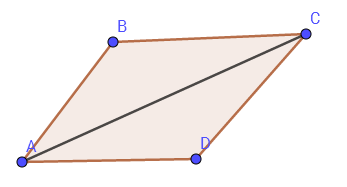这张是从网上盗的

即$AB+AD=AC$
复数相乘还有一个值得注意的小性质
$(a_1,\theta_1)*(a_2,\theta_2)=(a_1a_2,\theta_1+\theta_2)$
即模长相乘，极角相加

DFT（离散傅里叶变换）

一定注意从这里开始所有的$n$都默认为$2$的整数次幂

对于任意系数多项式转点值，当然可以随便取任意$n$个$x$值代入计算
但是暴力计算$x_k^0,x_k^1,...,x_k^{n-1}(k\in[0,n))$当然是$O(n^2)$的时间
其实可以代入一组神奇的$x$，代入以后不用做那么多的次方运算
这些$x$当然不是乱取的，而且取这些$x$值应该就是 傅里叶 的主意了
考虑一下，如果我们代入一些$x$，使每个$x$的若干次方等于$1$，我们就不用做全部的次方运算了
$±1$是可以的，考虑虚数的话$±i$也可以，但只有这四个数远远不够

傅里叶说：这个圆圈上面的点都可以做到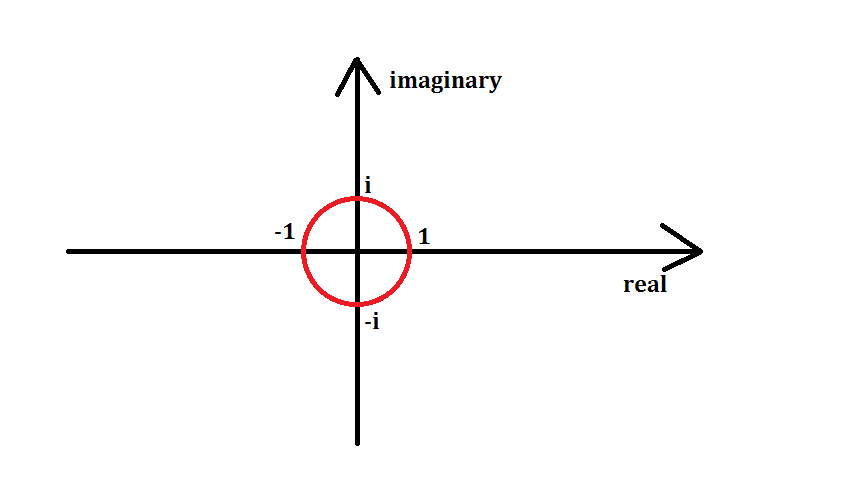以原点为圆心，画一个半径为$1$的单位圆
那么单位圆上所有的点都可以经过若干次次方得到$1$
傅里叶说还要把它给$n$等分了，比如此时$n=8$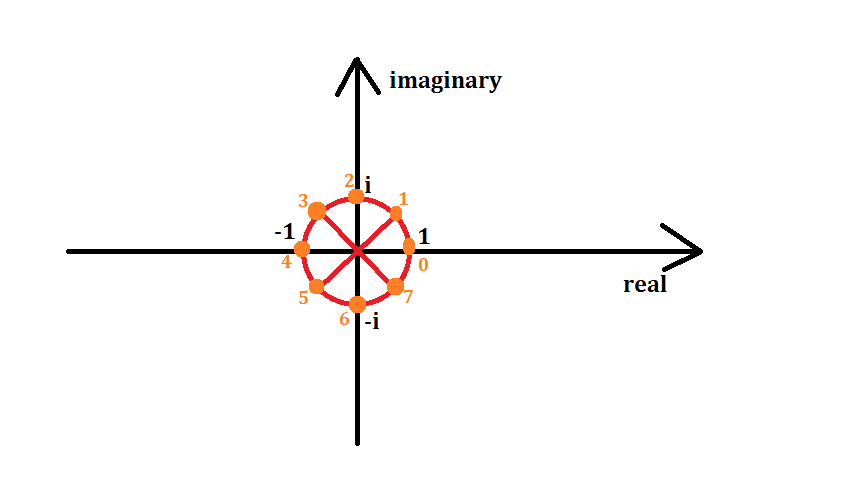橙色点即为$n=8$时要取的点，从$(1,0)$点开始，逆时针从$0$号开始标号，标到$7$号
记编号为$k$的点代表的复数值为$\omega_n^k$，那么由模长相乘，极角相加可知$(\omega_n^1)^k=\omega_n^k$
其中$\omega_n^1$称为$n$次单位根，而且每一个$\omega$都可以求出 （我三角函数不好）
$\omega_n^k=\cos{k\over n}2π+i\sin{k\over n} 2π$
或者说也可以这样解释这条式子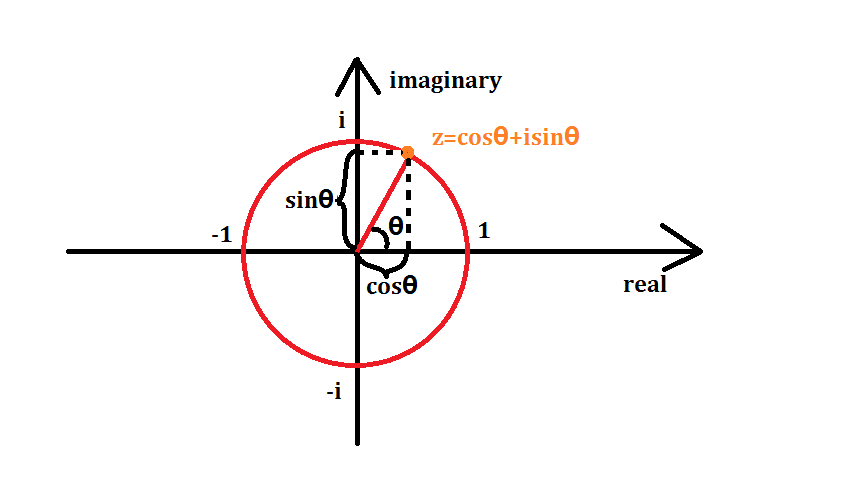注意$sin^2\theta+cos^2\theta=1$什么的，就容易理解了

那么$\omega^0_n,\omega^1_n,...,\omega^{n-1}_n$即为我们要代入的$x_0,x_1,...,x_{n-1}$

单位根的一些性质
推$FFT$的过程中需要用到$\omega$的一些性质

$\omega^k_n=\omega^{2k}_{2n}$

它们表示的点（或向量）表示的复数是相同的

证明

$\omega^k_n=cos{k\over n}2π+isin{k\over n} 2π=cos{2k\over 2n}2π+isin{2k\over 2n} 2π=\omega^{2k}_{2n}$

$\omega^{k+{n \over 2}}_n=-\omega_n^k$

它们表示的点关于原点对称，所表示的复数实部相反，所表示的向量等大反向

证明

$\omega^{n\over 2}_n=cos{{n\over 2}\over n}2\pi+isin{{n\over 2}\over n}2\pi=cos\pi+isin\pi=-1$

（这个东西和$e^{ix}=cosx+isinx$与$e^{i\pi}+1=0$有点关系，我不会就不讲了）

$\omega^0_n=\omega^n_n$

都等于$1$，或$1+0i$

FFT（快速傅里叶变换）
虽然$DFT$搞出来一堆很牛逼的$\omega$作为代入多项式的$x$值
但只是代入这类特殊$x$值法的变换叫做$DFT$而已，还是要代入单位根暴力计算

DFT还是暴力$O(n^2)$…

但$DFT$可以分治来做，于是 FFT（快速傅里叶变换） 就出来了
首先设一个多项式$A(x)$
$A(x)=\sum^{n-1}_{i=0}a_ix^i=a_0+a_1x+a_2x^2+...+a_{n-1}x^{n-1}$
按$A(x)$下标的奇偶性把$A(x)$分成两半，右边再提一个$x$
$A(x)=(a_0+a_2x^2+...+a_{n-2}x^{n-2})+(a_1x+a_3x^3+...+a_{n-1}x^{n-1})$
$=(a_0+a_2x^2+...+a_{n-2}x^{n-2})+x(a_1+a_3x^2+...+a_{n-1}x^{n-2})$
两边好像非常相似，于是再设两个多项式$A_1(x),A_2(x)$，令
$A_1(x)=a_0+a_2x+a_4x^2+...+a_{n-2}x^{{n\over 2}-1}$$A_2(x)=a_1+a_3x+a_5x^2+...+a_{n-1}x^{{n \over 2}-1}$
比较明显得出
$A(x)=A_1(x^2)+xA_2(x^2)$
再设$k<{n\over 2}$，把$\omega^k_n$作为$x$代入$A(x)$（接下来几步变换要多想想单位根的性质）
$A(\omega^k_n)=A_1((\omega^k_n)^2)+\omega^k_nA_2((\omega^k_n)^2)$$=A_1(\omega^{2k}_n)+\omega^k_nA_2(\omega^{2k}_n)=A_1(\omega^k_{n\over2})+\omega^k_nA_2(\omega^k_{n\over 2})$
那么对于$A(\omega^{k+{n\over2}}_n)$的话，代入$\omega^{k+{n \over 2}}_n$
$A(\omega^{k+{n\over 2}}_n)=A_1(\omega^{2k+n}_n)+\omega^{k+{n\over 2}}_nA_2(\omega^{2k+n}_n)$$=A_1(\omega^{2k}_n\omega^n_n)-\omega^k_nA_2(\omega^{2k}_n\omega^n_n)$$=A_1(\omega^{2k}_n)-\omega^k_nA_2(\omega^{2k}_n)=A_1(\omega^k_{n\over2})-\omega^k_nA_2(\omega^k_{n\over2})$

发现了什么？

$A(\omega^k_n)$和$A(\omega^{k+{n\over2}}_n)$两个多项式后面一坨东西只有符号不同
就是说，如果已知$A_1(\omega^k_{n\over 2})$和$A_2(\omega^k_{n\over 2})$的值，我们就可以同时知道$A(\omega^k_n)$和$A(\omega^{k+{n\over2}}_n)$的值
现在我们就可以递归分治来搞$FFT$了
每一次回溯时只扫当前前面一半的序列，即可得出后面一半序列的答案
$n==1$时只有一个常数项，直接$return$
时间复杂度$O(n\log_2n)$

IFFT（快速傅里叶逆变换）
想一下，我们不仅要会$FFT$，还要会IFFT（快速傅里叶逆变换）
我们把两个多项式相乘 （也叫求卷积），做完两遍$FFT$也知道了积的多项式的点值表示
可我们平时用系数表示的多项式，点值表示没有意义

怎么把点值表示的多项式快速转回系数表示法？

$IDFT$暴力$O(n^2)$做？其实也可以用$FFT$用$O(n\log_2n)$的时间搞

你有没有想过为什么傅里叶是把$\omega^k_n$作为$x$代入而不是别的什么数？
原因是有的但是有我也看不懂
由于我是沙雕所以只用记住一个结论

一个多项式在分治的过程中乘上单位根的共轭复数，分治完的每一项除以$n$即为原多项式的每一项系数

意思就是说$FFT$和$IFFT$可以一起搞

朴素版FFT板子
$c++$有自带的复数模板$complex$库
$a.real()$即表示复数$a$的实部
#include<complex>
#define cp complex<double>

void fft(cp *a,int n,int inv)//inv是取共轭复数的符号
{
if (n==1)return;
int mid=n/2;
static cp b[MAXN];
fo(i,0,mid-1)b[i]=a[i*2],b[i+mid]=a[i*2+1];
fo(i,0,n-1)a[i]=b[i];
fft(a,mid,inv),fft(a+mid,mid,inv);//分治
fo(i,0,mid-1)
{
cp x(cos(2*pi*i/n),inv*sin(2*pi*i/n));//inv取决是否取共轭复数
b[i]=a[i]+x*a[i+mid],b[i+mid]=a[i]-x*a[i+mid];
}
fo(i,0,n-1)a[i]=b[i];
}

两个多项式$a,b$相乘再转系数多项式$c$，通常只用打这么一小段
	cp a[MAXN],b[MAXN];
int c[MAXN];
fft(a,n,1),fft(b,n,1);//1系数转点值
fo(i,0,n-1)a[i]*=b[i];
fft(a,n,-1);//-1点值转系数
fo(i,0,n-1)c[i]=(int)(a[i].real()/n+0.5);//注意精度

很明显，FFT只能处理$n$为$2$的整数次幂的多项式
所以在$FFT$前一定要把$n$调到$2$的次幂
这个板子看着好像很优美，但是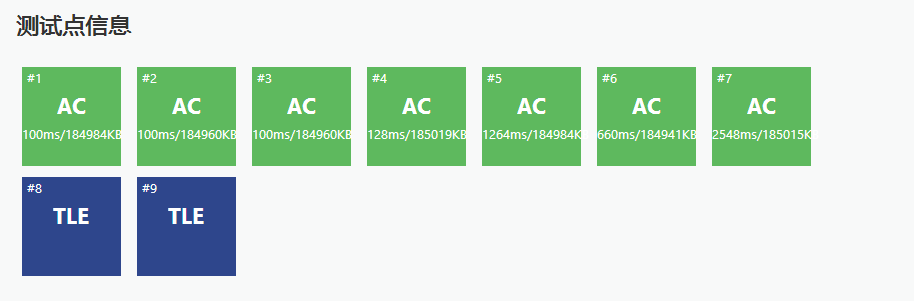递归常数太大，要考虑优化…

FFTの优化——迭代版FFT
这个图也是盗的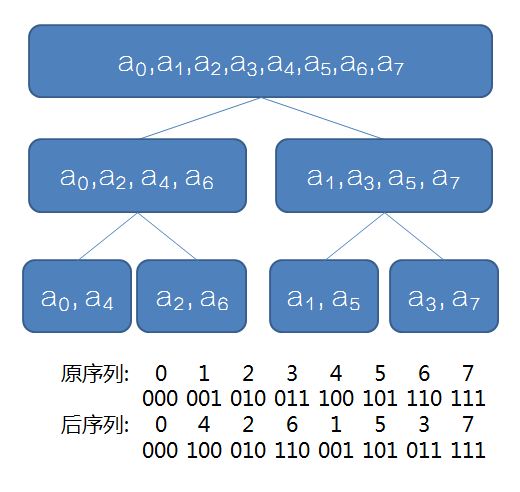这个很容易发现点什么吧？

每个位置分治后的最终位置为其二进制翻转后得到的位置

这样的话我们可以先把原序列变换好，把每个数放在最终的位置上，再一步一步向上合并
一句话就可以$O(n)$预处理第$i$位最终的位置$rev[i]$
fo(i,0,n-1)rev[i]=(rev[i>>1]>>1)|((i&1)<<(bit-1));

至于蝴蝶变换它死了其实是我不会

真·FFT板子
void fft(cp *a,int n,int inv)
{
int bit=0;
while ((1<<bit)<n)bit++;
fo(i,0,n-1)
{
rev[i]=(rev[i>>1]>>1)|((i&1)<<(bit-1));
if (i<rev[i])swap(a[i],a[rev[i]]);//不加这条if会交换两次（就是没交换）
}
for (int mid=1;mid<n;mid*=2)//mid是准备合并序列的长度的二分之一
{
cp temp(cos(pi/mid),inv*sin(pi/mid));//单位根，pi的系数2已经约掉了
for (int i=0;i<n;i+=mid*2)//mid*2是准备合并序列的长度，i是合并到了哪一位
{
cp omega(1,0);
for (int j=0;j<mid;j++,omega*=temp)//只扫左半部分，得到右半部分的答案
{
cp x=a[i+j],y=omega*a[i+j+mid];
a[i+j]=x+y,a[i+j+mid]=x-y;//这个就是蝴蝶变换什么的
}
}
}
}

这个板子好像不是那么好背
至少这个板子已经很优美了

FFT后记
本人版权意识薄弱……

本博客部分知识学习于

https://www.cnblogs.com/RabbitHu/p/FFT.html

https://www.cnblogs.com/zwfymqz/p/8244902.html?mType=Group#_label3

https://blog.csdn.net/ggn_2015/article/details/68922404

$NTT$我来了


展开全文• FFT——快速傅里叶变换 这块不写东西空荡荡的，我决定还是把FFT的定义给贴上吧 FFT（Fast Fourier Transformation）是离散傅氏变换（DFT）的快速算法。即为快速傅氏变换。它是根据离散傅氏变换的奇、偶、虚...
FFT——快速傅里叶变换
这块不写东西空荡荡的，我决定还是把FFT的定义给贴上吧

FFT（Fast Fourier Transformation）是离散傅氏变换（DFT）的快速算法。即为快速傅氏变换。它是根据离散傅氏变换的奇、偶、虚、实等特性，对离散傅立叶变换的算法进行改进获得的。

这三段话其实一点用也没有
FFT是干什么的
FFT在算法竞赛中就有一个用途：加速多项式乘法(暴言)
简单来说，形如 $a_0X^0+a_1X^1+a_2X^2+⋯+a_nX^n$ 的代数表达式叫做多项式，可以记作$f(X)=a_0X^0+a_1X^1+a_2X^2+⋯+a_nX^n$，其中$a_0,a_1,⋯,a_n$叫做多项式的系数，$X$是一个不定元(就是不可以合并)，不表示任何值，不定元在多项式中最大项的次数称作多项式的次数
如果我们当前有两个多项式$f(X),g(X)$，现在要把他们乘起来(求卷积)，最朴素的做法就是
$\sum \limits _{i=0}^{2n-1} (\sum \limits _{j=0} ^{i} a_j*b_{i-j})*x^i$
这样的复杂度是$\varTheta(n^2)$的，十分不美观，FFT就是要将这个过程优化为$\varTheta(n \log n)$
前置技能
多项式
见上文
复数
复数形如$a+bi$，其中$i=\sqrt {-1}$
$a$叫作复数的实部，$bi$叫做复数的虚部
复数$(a_1+b_1i)*(a_2+b_2i)$相乘的值，即$a_1a_2-b_1b_2+(a_1b_2+a_2b_1)i$也是一个复数，同时我们也得到了复数的乘法法则
复数$c+di$可以用这种方式表示出来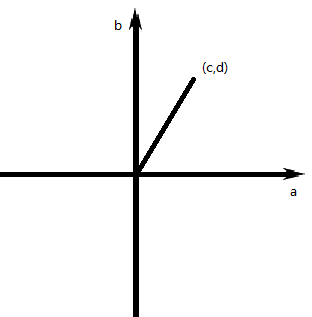复数乘法的在复平面中表现为辐角相加，模长相乘
单位根
复数$w$满足$w^n=1$称作$w$是$n$次单位根，下图包含了所有的$8$次单位根(图中圆的半径是1)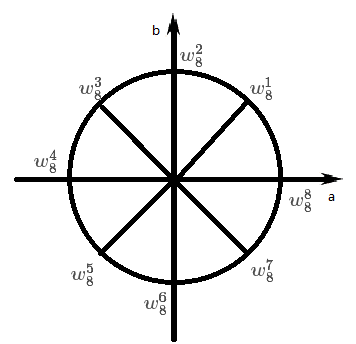同样的，下图是所有的4次单位根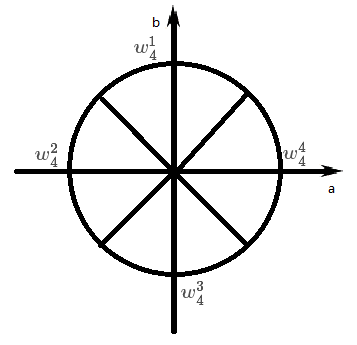聪明的你也许已经发现了单位根的些许性质，即
$w_{2n}^{2m}=w_{n}^{m}$
$w_n^m=-w_n^{m+\frac{n}{2}}$
这两个要记住，一会很有用
多项式的系数表达法
我们有多项式$f(X)=a_0X^0+a_1X^1+a_2X^2+⋯+a_nX^n$，令$\vec{a}=(a_0,a_1,a_2,...,a_n)$，则称$A(X)$为多项式$f(X)$的系数表示法
在系数表示法下，计算多项式乘法是$\varTheta (n^2)$的
多项式的点值表达法
任取$n+1$个互不相同的$S=\{p_1,p_2,...,p_{n+1}\}$，对$f(X)$分别求值得到$f(p_1),f(p_2),...,f(p_{n+1})$，那么称$A(X)=\{(p_1,f(p_1)),(p_2,f(p_2)),...,(p_{n+1},f(p_{n+1}))\}$为多项式$f(X)$在$S$下的点值表示法
可以把多项式想象成一个$n$次函数，点值表示法就是取$S$下每一个横坐标时对应的点，因为$n$次函数可以由$n+1$个点确定下来(可以将每一个点列一个$n$次方程)，所以$n$维点值与$n$维系数一一对应
更重要的一点，点值表示法下的乘法运算获得了简化
两个多项式$P$,$Q$分别取点$(x,y_1)$和$(x,y_2)$，$P*Q$就会取到点$(x,y_1*y_2)$；
令$C=P*Q$，因为$C(X)=P(X)*Q(X)$，所以$C(x)=P(x)*Q(x)$，即$C(x)=y_1*y_2$
所以在点值表示法下，计算多项式乘法是$\varTheta(n)$的
FFT的具体过程
FFT就是将系数表示法转化成点值表示法相乘，再由点值表示法转化为系数表示法的过程，第一个过程叫做求值(DFT)，第二个过程叫做插值(IDFT)
求值
还记得我们之前提到的单位根吗？再回顾一下：
$w_{2n}^{2m}=w_{n}^{m}$
$w_n^m=-w_n^{m+\frac{n}{2}}$
想要求出一个多项式的点值表示法，需要选出$n+1$个数分别带入到多项式里面，带入一个数的复杂度是$\varTheta(n)$的，那么总复杂度就是$\varTheta(n^2)$的，因为单位根有上面两个优美的性质，所以我们尝试可以取$n$次单位根组成$S$，看看能不能加速我们的运算
设$A_0(X)$为$A(X)$偶次项的和，设$A_1(X)$为$A(X)$奇次项的和，即
$A_0(X)=a_0x^0+a_2x^1+...+a_{n-1}x^{n/2}$
$A_1(X)=a_1x^0+a_3x^1+...+a_{n-2}x^{n/2}$
因为$A(w_n^m)=a_0w_n^0+a_1w_n^m+a_2w_n^{2m}+a_3w_n^{3m}+...+a_{n-1}w_n^{(n-1)*m}+a_nw_n^{nm}$
所以有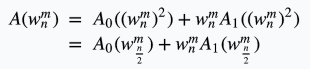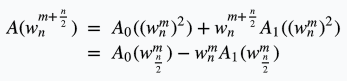也就是说，只要有了$A_0(X)$和$A_1(X)$的点值表示，就能在$\varTheta(n)$时间算出$A(X)$的点值表示，对于当前层确定的位置$i$，就可以用下一层的两个值更新当前的值，我们称这个操作为“蝴蝶变换”
因为这个过程一定要求每层都可以分成两大小相等的部分，所以多项式最高次项一定是$2^p$($p\in N$)次方，如果不是的话，直接在最高次项补零就可以啦
于是我们有了递归的写法：
void FFT(Complex* a,int len){
if(len==1) return;
Complex* a0=new Complex[len/2];
Complex* a1=new Complex[len/2];
for(int i=0;i<len;i+=2){
a0[i/2]=a[i];
a1[i/2]=a[i+1];
}
FFT(a0,len/2);FFT(a1,len/2);
Complex wn(cos(2*Pi/len),sin(2*Pi/len));
Complex w(1,0);
for(int i=0;i<(len/2);i++){
a[i]=a0[i]+w*a1[i];
a[i+len/2]=a0[i]-w*a1[i];
w=w*wn;
}
return;
}

但递归版的FFT常数巨大，实现起来比较复杂，于是又有了迭代的写法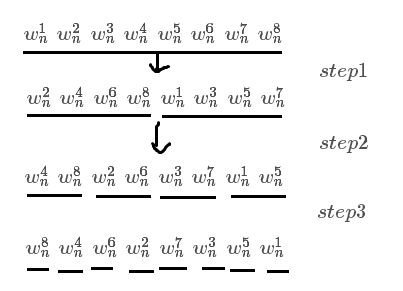重新考虑下递归FFT的过程，在第$i$次求解中，我们将所有元素二进制$i$位为$0$的放在了左面，$i$位为$1$的放在了右面，事实上，每个元素最终到的是他二进制颠倒过来的位置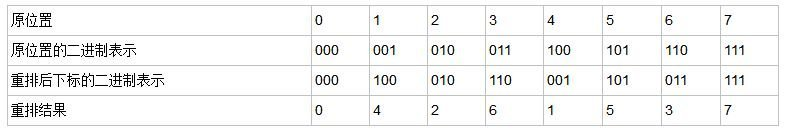再拿一张别人的图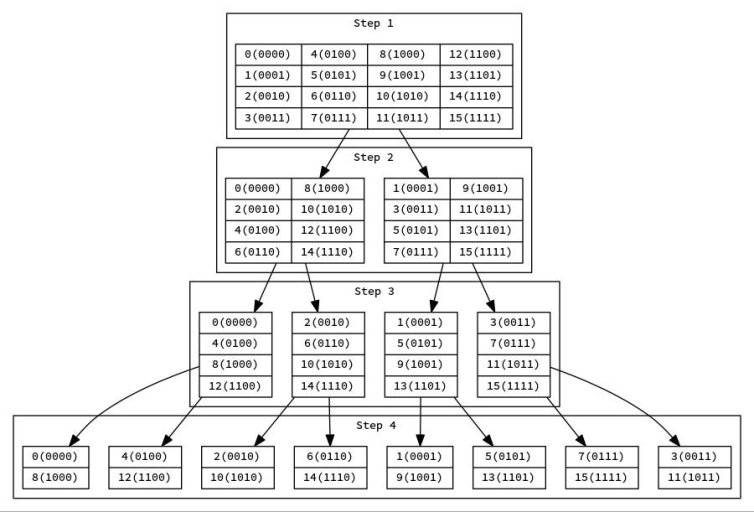迭代写法：
inline void DFT(Complex a[]){
for(int i=0;i<len;i++)///pos[i]代表反转后的位置
if(i<pos[i])
swap(a[i],a[pos[i]]);
for(int i=2,mid=1;i<=len;i<<=1,mid<<=1){///len代表多项式最高次项
///第一层i是枚举合并到了哪一层。
Complex wm(cos(2.0*pi/i),sin(2.0*pi/i));
for(int j=0;j<len;j+=i){///第二层j是枚举合并区间。
Complex w(1,0);
for(int k=j;k<j+mid;k++,w=w*wm){///第三层k是枚举区间内的下标。
Complex l=a[k],r=w*a[k+mid];
a[k]=l+r;a[k+mid]=l-r;
}
}
}
return;
}

插值
有人证出来插值只要将所有$w_n^m$换成$w_n^{m+\frac{n}{2}}$，也就是所有的虚部取相反数，再将最终结果除以$len$，就是插值的过程了
究竟为什么？我觉得人生一定要有点遗憾可以参考这里，我就不多说了
支持插值的迭代写法：
const double DFT=2.0,IDFT=-2.0;///进行求值，第二个参数传DFT，插值传IDFT
inline void FFT(Complex a[],double mode){
for(int i=0;i<len;i++)
if(i<pos[i])
swap(a[i],a[pos[i]]);
for(int i=2,mid=1;i<=len;i<<=1,mid<<=1){
Complex wm(cos(2.0*pi/i),sin(mode*pi/i));
for(int j=0;j<len;j+=i){
Complex w(1,0);
for(int k=j;k<j+mid;k++,w=w*wm){
Complex l=a[k],r=w*a[k+mid];
a[k]=l+r;a[k+mid]=l-r;
}
}
}
if(mode==IDFT)
for(int i=0;i<len;i++)
a[i].x/=len;
return;
}

现在，你已经会写FFT了！
生成函数

小$A$有$a_i$个价值为$A_i$的物品，小$B$有$b_i$个价值为$A_i$的物品，求用两个组成价值为$c_i$的方案数

生成函数可以解决上面的这个问题，构造两个多项式，第$i$项表示价值为$i$的物品有多少个，对两个人分别构造，乘在一起的多项式就代表所有的方案数了
NTT——快速数论变换
当FFT需要取模时，复数的存在就特别尴尬了，有没有什么东西可以代替单位根呢？
原根性质：假设$g​$是素数$p​$的一个原根，则$g^1,g^2,...,g^{(p-1)}​$在模$p​$意义下两两不同，且结果恰好为$1​$~$p-1​$
$w_n^n≡g^{(p-1)}≡1(mod\ p)$(中间那个是费马小定理)
所以可以把$g^{(p-1)/n}$看成$w_n^1$的等价。
但这种质数必须是NTT质数(费马质数)，即$(p-1)$有超过序列长度的2的正整数幂因子的质数
具体证明还是看这里吧
参考资料：
Pick’s blog
Fenghr的博客
Miskcoo’s Space


展开全文• ## 浅谈 FFT （终于懂一点了~~）

万次阅读 多人点赞 2019-10-25 23:06:28
FFT（离散傅氏变换的快速算法） FFT（Fast Fourier Transformation）是离散傅氏变换（DFT）的快速算法。即为快速傅氏变换。它是根据离散傅氏变换的奇、偶、虚、实等特性，对离散傅立叶变换的算法进行改进获得的。 ...
FFT（离散傅氏变换的快速算法）

FFT（Fast Fourier Transformation）是离散傅氏变换（DFT）的快速算法。即为快速傅氏变换。它是根据离散傅氏变换的奇、偶、虚、实等特性，对离散傅立叶变换的算法进行改进获得的。

以上内容摘自百度百科,其实看了等于没看

首先先要知道一些预备知识：
1、多（door♂）项式

2、复数的运算（不是负数）

3、下面开始讲吧……

首先，多（door♂）项式是蛤？

也可表示为

这个就是多项式，一般是吧高次项写在前面，我这里这样写只是为了方便。

那么FFT是用来干蛤的？

这个问题问得好，FFT是用来算两个多项式相乘的。

那不可以暴力乘吗（）？

那暴力可以做到  吗？

。。。。

---------------------------------------------------------------------------------先分个鸽-------------------------------------------------------------------------------------

首先先要有复数运算的知识

我们知道  （是个虚数）

那么对于形如，其中a,b为实数，i为虚数的东东我们称它为复数

a 为实部，bi为虚部。

加法运算： 实部+实部 虚部+虚部

减法运算：同上，‘+’ —> ‘-’

乘法运算：就和正常的多项式乘法差不多，只不过要注意

除法运算：分数上下同时乘分母的共轭

即：

一个复数共轭就是一个实部相同，虚部为相反数的复数

如的共轭是

一个复数的共轭通常表示为

可得

因为所以分母必定为实数

对于  我们还可以这样子表示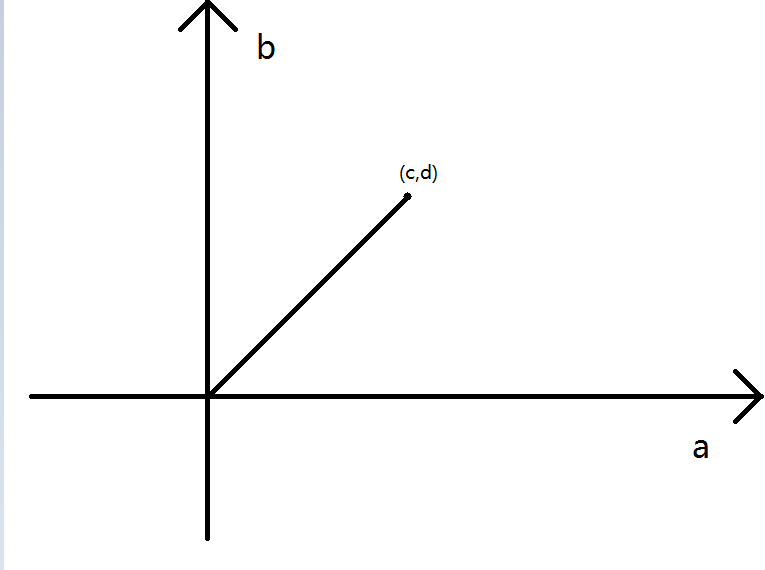其中a为实轴，b为虚轴， 就可以表示为在这样一个二维平面上的一个点

复数乘法在平面中就是辐角相加，模长相乘  （这个用三角函数可以证明，这里就不多说了）

真香

证明：对于可以表示为其中为辐角,为半径（即模长），然后相乘就直接r相乘指数相加就行了

-----------------------------------------------------------------------------再次分鸽----------------------------------------------------------------------------------

下面重点来辣！重点警告！！重点警告！！重点警告！！（重要的事情说三遍（滑稽

嗯哼。。。。好了继续

然后是一个很重要的东西

单位根

首先这是个圆（废话），它的半径是一，所以称为单位圆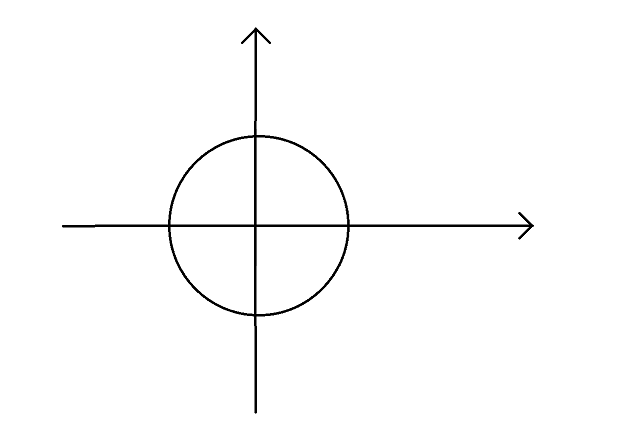复数满足称作是次单位根

如当是，w可以为：,,.

在平面上表示的话……算了吧

真香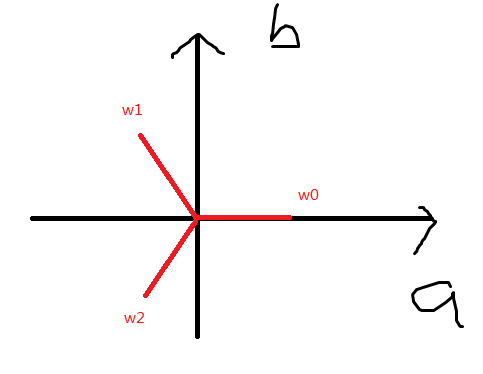即将圆等分成3份

n次单位根就是将单位圆等分成n份

一下记第k个n次单位根为（从1开始，逆时针第k+1个为）

单位根的特殊性质可以保证这n-1个复数各不相等

单位根还有一些性质：

(高清无码）

这两条性质带进下面的欧拉公式就可以算出来了

对了忘记说怎么算了，用我们强dark的欧拉公式可以解决：

欧拉公式：

因为c++是用弧度制所以我下面也用弧度制表示(即表示,表示)

-----------------------------------------------------------------------------------分鸽------------------------------------------------------------------------------------

现在可以开始讲了

（当当当当）

还记得这个东东吗，不过这只是多项式的一种表达方式，叫作：系数表达法

还有种表达法叫：

点值表达法

设

我们知道，n+1个互不相同的点就可以确定一个n次函数，对分别求值就可以得到,那么称为door项式的点表示法

---------------------------------------------------------------------------啦啦啦啦啦啦啦啦（还是分鸽）-----------------------------------------------------------

为蛤要用点值表示法呢？

因为我们现，系数表示法算多项式乘法的时间复杂度是,而通过点值表示法我们可以发现两个多项式,同时取点时，得到的是和,即取到的点分别为而会取到的点为（显而易见）。那么计算的点表达式的时间复杂度就是的。

我知道了o(*^▽^*)┛，就是把值直接带进去，然后就可以线性求出来了，干嘛要啊？

但是……你要带个值进去才可以弄出点表示法呀。

.........那不还是的吗，哪来的...

有办法让这个过程变成,别急，马上就要讲了。

-------------------------------------------------------------------------分鸽（下面就不解释了）------------------------------------------------------------------------

FFT就是将系数表示法转化成点值表示法相乘，再由点值表示法转化为系数表示法的过程，第一个过程叫做求值(DFT)，第二个过程叫做插值(IDFT)——摘自一位darklao的blog

首先是求值DFT

(高清无码++）

再次把单位根的性质搬出来。。

想要求出一个多项式的点值表示法，需要选出个数分别带入到多项式里面，带入一个数的复杂度是的，那么总复杂度就是的，因为单位根有上面两个优美的性质，所以我们尝试可以取次单位根组成，看看能不能加速我们的运算....

设为X的指数为偶数次的，为X的指数为奇数次的

可得

(这里n为2^k,不够就补齐，反正系数为0，不影响）

然后我们发现和是两个长度为原来一半的多项式,然后就可以分治了

把单位根带进去

由上面的性质可化简得

和递归进去算就行了，然后就先把算出来，然后k次幂就行了

儿当就不管用了，因为单位根出现了重复

先把式子列出来

用上面的性质化简一下就变成

然后就可以愉快地把系数表达式转换为点值表达式

||||||好了，求值（DFT）搞定了，下面是插值。||||||

-------------------------------------------------------------分鸽线（真香*2）--------------------------------------------------------------------

插值只要将所有的变成,就是将虚部取反，再将结果除以长度（即n），至于为甚，我们可以这样考虑。

我们可以考虑把原来的要做的操作用矩阵的形式表现出来：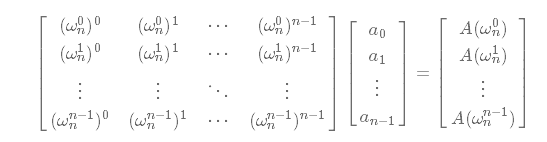这是DFT,我们要求的IDFT，我们已知了左边和右边的两个矩阵，要求中间那个，就相当于是求最左边那个矩阵的逆，然后乘右边那个矩阵。它的逆就是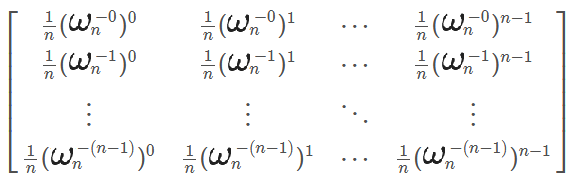这个手推一下就行了，是因为带入了n个值。然后发现IDFT和DFT差不多

(下面的推导要用到  和   )

然后就可以了。

真香……

递归的写法：

#include<bits/stdc++.h>
#define N 8000005
using namespace std;
const double pi = acos(-1.0);
const double eps = 1e-6;
struct complexx{
double x, y;
complexx(double xx = 0, double yy = 0) {x = xx, y = yy;}
}a[N], b[N];
complexx operator + (complexx a, complexx b) {return complexx(a.x + b.x, a.y + b.y);}
complexx operator - (complexx a, complexx b) {return complexx(a.x - b.x, a.y - b.y);}
complexx operator * (complexx a, complexx b) {return complexx(a.x * b.x - a.y * b.y, a.y * b.x + a.x * b.y);}
void fft(int len, complexx *a, int o){
if(len == 1) return;
complexx a0[(len >> 1) + 3], a1[(len >> 1) + 3];
for(int i = 0; i <= len; i += 2)
a0[i >> 1] = a[i], a1[i >> 1] = a[i + 1];
fft(len >> 1, a0, o);
fft(len >> 1, a1, o);
complexx wn = complexx(cos(2 * pi / len), o * sin(2 * pi / len)), w0 = complexx(1, 0);
for(int i = 0; i < (len >> 1); i ++, w0 = w0 * wn){
a[i] = a0[i] + w0 * a1[i];
a[i + (len >> 1)] = a0[i] - w0 * a1[i];
}
}
int n, m;
int main(){
scanf("%d%d", &n, &m);
for(int i = 0; i <= n; i ++) scanf("%lf", &a[i].x);
for(int i = 0; i <= m; i ++) scanf("%lf", &b[i].x);
int len = 1;
for(;len <= n + m; len <<= 1);
fft(len, a, 1);//DFT
fft(len, b, 1);//DFT
for(int i = 0; i <= len; i ++)
a[i] = a[i] * b[i];
fft(len, a, -1);//IDFT
for(int i = 0; i <= n + m; i ++) printf("%.0f ", a[i].x / len + eps);//记得除len
return 0;
}

----------------------------------------------------------------------------分个鸽（真香）--------------------------------------------------------------------

当然，递归的写法常数巨dark,于是乎就有了非递归即迭代的写法。

先盗个图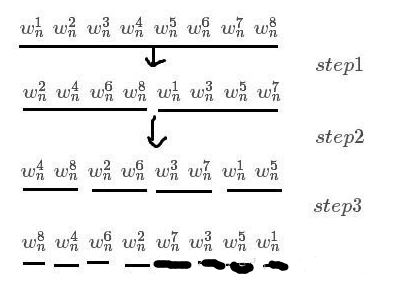当我们做递归版的FFT的时候，在第i层就相当于把当前数的二进制第i位为零的分一类，为一的分一类，然后分别递归进去。

再偷一张图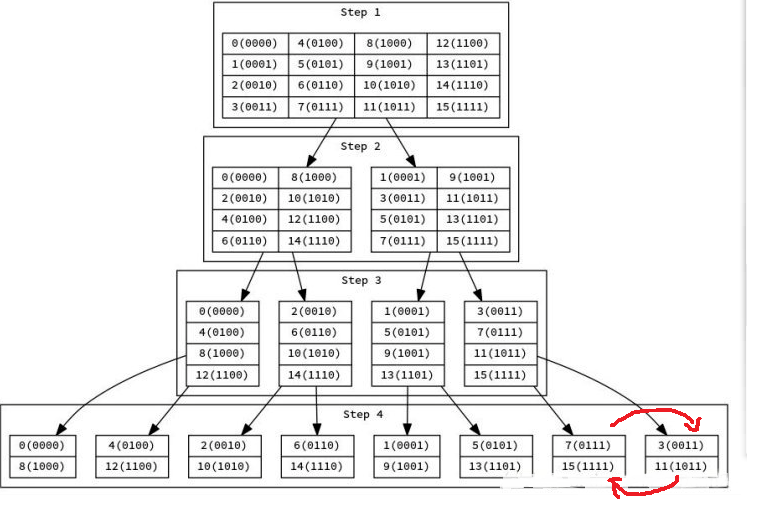注意这副图的原图最后两个地方放反了

现在应该清楚很door了吧。

那么代码：（待填坑……）

等等（update 2019.4.9）

时隔将近一年，终于来填坑了，看看之前写的，感觉初一的时候还是太菜（现在还是很菜）。

emmmmmm……

讲一蛤fft的优化吧

首先看之前的代码，发现算三角函数的那部分被重复调用了很多次，是没有必要的，所以可以先预处理。

设的实部为，虚部为,

即

由

可得

然后把虚部多除个2就是答案了

code：

#include<bits/stdc++.h>
#define N 8000005
using namespace std;
const double pi = acos(-1.0);
struct complexx{
double x, y;
complexx(double xx = 0, double yy = 0) {x = xx, y = yy;}
}a[N];
double coss[N], sinn[N];
complexx operator + (complexx a, complexx b) {return complexx(a.x + b.x, a.y + b.y);}
complexx operator - (complexx a, complexx b) {return complexx(a.x - b.x, a.y - b.y);}
complexx operator * (complexx a, complexx b) {return complexx(a.x * b.x - a.y * b.y, a.y * b.x + a.x * b.y);}
void fft(int len, complexx *a, int o){
if(len == 1) return;
complexx a0[(len >> 1) + 3], a1[(len >> 1) + 3];
for(int i = 0; i <= len; i += 2)
a0[i >> 1] = a[i], a1[i >> 1] = a[i + 1];
fft(len >> 1, a0, o);
fft(len >> 1, a1, o);
complexx wn = complexx(coss[len], o * sinn[len]), w0 = complexx(1, 0);
for(int i = 0; i < (len >> 1); i ++, w0 = w0 * wn){
a[i] = a0[i] + w0 * a1[i];
a[i + (len >> 1)] = a0[i] - w0 * a1[i];
}
}
int n, m;
int main(){
scanf("%d%d", &n, &m);
for(int i = 0; i <= n; i ++) scanf("%lf", &a[i].x);
for(int i = 0; i <= m; i ++) scanf("%lf", &a[i].y);
int len = 1;
for(;len <= n + m; len <<= 1);
for(int i = 1; i <= len; i <<= 1) coss[i] = cos(2 * pi / i), sinn[i] = sin(2 * pi / i);//预处理三角函数，只用处理2^k的
fft(len, a, 1);
for(int i = 0; i <= len; i ++)
a[i] = a[i] * a[i];
fft(len, a, -1);
for(int i = 0; i <= n + m; i ++) printf("%.0f ", a[i].y / 2 / len + 0.49);
return 0;
}

好像快了一点，但是仅限如此吗？

现在来填坑了！！！

蝴蝶变换还是先看这张图

原序列：0 1 2 3 4 5 6 7 8 9 10 11 12 13 14 15

位逆序置换后：0 8 4 12 2 10 6 14 1 9 5 13 3 11 7 15

然后我们发现了一个神奇的规律，把原序列和置换后的序列的对应位转换为二进制之后是刚好翻转过来的

如1的二进制是0001，8的二进制则是1000，2的二进制是0010，4的二进制是0100，其他也都是如此

证明也十分简单，手推一下就可以了

然后按照置换后的一层一层往上合并就行了

位逆序置换可以用类似数位dp的方法求出来

code：

#include<bits/stdc++.h>
#define N 8000005
using namespace std;
const double pi = acos(-1.0);
struct complexx{
double x, y;
complexx(double xx = 0, double yy = 0) {x = xx, y = yy;}
}a[N];
double coss[N], sinn[N];
int rev[N];
complexx operator + (complexx a, complexx b) {return complexx(a.x + b.x, a.y + b.y);}
complexx operator - (complexx a, complexx b) {return complexx(a.x - b.x, a.y - b.y);}
complexx operator * (complexx a, complexx b) {return complexx(a.x * b.x - a.y * b.y, a.y * b.x + a.x * b.y);}
void fft(int len, complexx *a, int o){

for(int i = 0; i <= len; i ++) if(i < rev[i]) swap(a[i], a[rev[i]]);
for(int j = 1; j < len; j <<= 1){//j枚举的是合并区间长度的一半，即把两个长度为j的序列合成一个长度为2*j的序列
complexx wn = complexx(coss[j], o * sinn[j]);
for(int k = 0; k < len; k += (j << 1)){//k为当前处理的区间的开头
complexx w0 = complexx(1, 0);
for(int i = 0; i < j; i ++, w0 = w0 * wn){//i为对应位
complexx X = a[i + k], Y = w0 * a[i + j + k];
a[i + k] = X + Y;
a[i + k + j] = X - Y;//合并
}
}
}
}
int n, m;
int main(){
scanf("%d%d", &n, &m);
for(int i = 0; i <= n; i ++) scanf("%lf", &a[i].x);
for(int i = 0; i <= m; i ++) scanf("%lf", &a[i].y);
int len = 1, l = 0;
for(;len <= n + m; len <<= 1, l ++);
for(int i = 0; i <= len; i ++) rev[i] = (rev[i >> 1] >> 1) | ((i&1) << (l - 1));//rev[i]位将i按二进制后的值（类似数位dp的思想）
for(int i = 1; i <= len; i <<= 1) coss[i] = cos(pi / i), sinn[i] = sin(pi / i);//注意这里pi不用乘2
fft(len, a, 1);
for(int i = 0; i <= len; i ++)
a[i] = a[i] * a[i];
fft(len, a, -1);
for(int i = 0; i <= n + m; i ++) printf("%.0f ", a[i].y / 2 / len + 0.49);
return 0;
}

FFT的运用！！

前面哔了那么多，可fft除了求多项式乘法还有什么用呢？

没什么用了

因为所有的卷积都可以表示为多项式乘法的形式，所以求卷积的时候之久用fft求就可以了。

卷积

定义：

设 ,  是两个数列，那么这两个数列的卷积  的定义为

可以很容易的看出多项式乘法和卷积是类似的

设

那么

所以遇到要求卷积的时候直接用法法踢就可以了

练习题：https://blog.csdn.net/qq_38944163/article/details/89145308 卷积

https://www.luogu.org/problemnew/show/P1919 多项式乘法

https://www.luogu.org/problemnew/show/P4199万径人踪灭

https://www.luogu.org/problemnew/show/P4173残缺的字符串

https://www.luogu.org/problemnew/show/P3763 [TJOI2017]DNA

https://www.luogu.org/problemnew/show/P3723[AH2017/HNOI2017]礼物

https://www.luogu.org/problemnew/show/P3338[ZJOI2014]力

参考资料：FFT算法讲解——麻麻我终于会FFT了！  （通俗易懂，感谢ing）

从多项式乘法到快速傅里叶变换（精准有用，感谢ing）

最后附一句：不要问我是怎么在不用markdown的情况下写下这篇文章的！！！

展开全文• ## FFT详解

千次阅读 2019-03-24 16:24:22
matlab 中fft的用法 一.调用方法 X=FFT(x)； X=FFT(x，N)；x=IFFT(X);x=IFFT(X,N) 用MATLAB进行谱分析时注意： （1）函数FFT返回值的数据结构具有对称性。 例： N=8; n=0:N-1; xn=[4 3 2 6 7 8 9 0]; Xk=fft(xn) →...
• 原图链接：（！！！） 转载于:https://www.cnblogs.com/zpfbuaa/p/5099680.html
• 著名的多项式练习题，做法也很多，终于切掉了纪念 首先求一波递推式: 令$$F(n)$$为$$n$$个点的有标号无向连通图的个数，则考虑补集转化为有标号无向不连通图的个数，然后枚举$$1$$号点所在联通块的大小: \[F(n)=2^{n...
• ## FFT

千次阅读 多人点赞 2018-04-11 10:04:56
FFT是离散傅立叶变换的快速算法，可以将一个信号变换到频域。有些信号在时域上是很难看出什么特征的，但是如果变换到频域之后，就很容易看出特征了。这就是很多信号分析采用FFT变换的原因。另外，FFT可以将一个信号...
• ## FFT在matlab中的使用方法

万次阅读 多人点赞 2020-07-10 14:44:50
FFT在matlab中的用法 一、FFT的物理意义 ​ FFT是离散傅立叶变换的快速算法，可以将一个信号变换到频域。有些信号在时域上是很难看出什么特征的，但是如果变换到频域之后，就很容易看出特征了。这就是很多信号分析...
• ## fft

2011-06-10 19:15:00
FFT是离散傅立叶变换的快速算法，可以将一个信号变换到频域。有些信号在时域上是很难看出什么特征的，但是如果变换到频域之后，就很容易看出特征了。这就是很多信号分析采用FFT变换的原因。另外，FFT可以将一个信号...plot matlab 算法 测试
• 以前一直对MATLAB中fft（）函数的使用一直存在疑惑，为什么要加一 些参数，并且如何确定这些参数，也查了许多资料，但很多都感觉只是 表面一说根本没有讲清其本质。但随着学习的推进，慢慢有所领悟，所 以打算把...MATLAB
• 最近，因为项目需要在STM32F103系列处理器上，对采集的音频信号进行FFT运算，然而STM32F103毕竟不是STM32F4系列的处理器，对于一般的FFT运算程序还是比较缓慢的。 幸亏官方提供了针对FFT的官方库，但是去官网找了...STM32 STM32F103 音频处理
• 快速傅里叶变换（FFT）说到FFT，我们就不得不介绍傅里叶同学、傅里叶级数、傅里叶变换、离散傅里叶变换。下面对他们这些玩意一一介绍。 1.傅里叶同学简介让·巴普蒂斯·约瑟夫·傅里叶（Baron Jean Baptiste Joseph...快速傅里叶变换 离散傅里叶变换 傅里叶变换
• ## 我所理解的快速傅里叶变换（FFT）

万次阅读 多人点赞 2016-10-28 14:41:26
首先FFT是离散傅立叶变换(DFT)的快速算法，那么说到FFT，我们自然要先讲清楚傅立叶变换。先来看看傅立叶变换是从哪里来的？ 傅立叶是一位法国数学家和物理学家的名字，英语原名是Jean Baptiste Joseph Fourier(1768...
• ## matlab信号频谱分析FFT详解

万次阅读 多人点赞 2019-06-13 09:24:38
前言 做OFDM通信少不了频谱分析，基带信号DA后的频谱，以及基带数字上变频后的DA信号都要频谱分析。我觉得其实做任何工程都是这样，先规定实施方案，然后仿真成功...matlab使用FFT函数分析信号频谱 一般我使用的F...MATLAB 频谱分析
• FFT 前言：感谢M哥,Pls,Zjq等等一系列大神的指导，让我大概了解了FFT的基本原理以及能做的事情，下面是FFT的一些简单入门题（我也只会这些题，在这里总结一下。 由于目前题量较少，对于我目前的理解，FFT主要解决...
• 近期要对一款高速ADC进行测试，用到Matlab的fft函数分析其动态性能，为了对Matlab 的fft有一个全方位立体的认识，对其参数进行了小实验，记录如下。 使用Matlab生成采样数据 clear; fs = 1000; ts = 1/fs; L = ...
• 陆续会更新，包括FFT、巴特沃斯滤波器（高低带通、高低带阻）、数据差值（线性、sinc、三次样条*）、数据压缩（等距、平均、峰值检测）和模仿matlab的STFT功能（spectrogram函数三维绘图）。 注：可能会有不足...ios开发 matlab
...